Download Presentation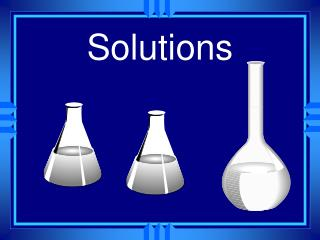Solutions

# Solutions - PowerPoint PPT Presentation

Solutions. Occur in all phases. The solvent does the dissolving. The solute is dissolved. There are examples of all types of solvents dissolving all types of solutes. We will focus on aqueous solutions. Ways of Measuring. Molarity = moles of solute Liters of solutionI am the owner, or an agent authorized to act on behalf of the owner, of the copyrighted work described.
Download Presentation## Solutions

An Image/Link below is provided (as is) to download presentation

Download Policy: Content on the Website is provided to you AS IS for your information and personal use and may not be sold / licensed / shared on other websites without getting consent from its author.While downloading, if for some reason you are not able to download a presentation, the publisher may have deleted the file from their server.

- - - - - - - - - - - - - - - - - - - - - - - - - - E N D - - - - - - - - - - - - - - - - - - - - - - - - - -
Presentation Transcript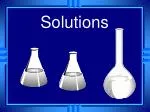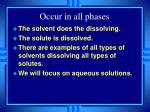Occur in all phases
• The solvent does the dissolving.
• The solute is dissolved.
• There are examples of all types of solvents dissolving all types of solutes.
• We will focus on aqueous solutions.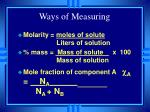Ways of Measuring
• Molarity = moles of solute Liters of solution
• % mass = Mass of solute x 100 Mass of solution
• Mole fraction of component A cA = NANA + NB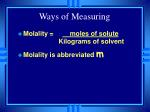Ways of Measuring
• Molality = moles of solute Kilograms of solvent
• Molality is abbreviated m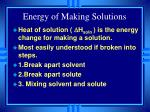Energy of Making Solutions
• Heat of solution ( DHsoln ) is the energy change for making a solution.
• Most easily understood if broken into steps.
• 1.Break apart solvent
• 2.Break apart solute
• 3. Mixing solvent and solute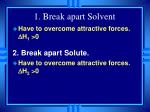1. Break apart Solvent
• Have to overcome attractive forces. DH1 >0

2. Break apart Solute.

• Have to overcome attractive forces. DH2 >0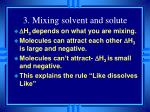3. Mixing solvent and solute
• DH3 depends on what you are mixing.
• Molecules can attract each other DH3 is large and negative.
• Molecules can’t attract- DH3 issmall and negative.
• This explains the rule “Like dissolves Like”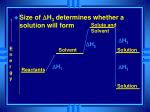Solute and Solvent

DH3

DH2

Solvent

DH1

DH3

Solution

Solution

• Size of DH3 determines whether a solution will form

Energy

Reactants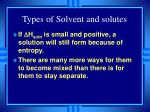Types of Solvent and solutes
• If DHsoln is small and positive, a solution will still form because of entropy.
• There are many more ways for them to become mixed than there is for them to stay separate.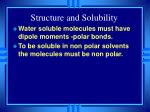Structure and Solubility
• Water soluble molecules must have dipole moments -polar bonds.
• To be soluble in non polar solvents the molecules must be non polar.O-

P

O-

CH2

CH2

CH2

CH3

CH2

O-

CH2

CH2

CH2

Soap
• Hydrophobic non-polar endO-

P

O-

CH2

CH2

CH2

CH3

CH2

O-

CH2

CH2

CH2

Soap
• Hydrophilic polar endA drop of grease in water
• Grease is non-polar
• Water is polar
• Soap lets you dissolve the non-polar in the polar.Water molecules can surround and dissolve grease.
• Helps get grease out of your way.Pressure effects
• Changing the pressure doesn’t effect the amount of solid or liquid that dissolves
• They are incompressible.
• It does effect gases.Dissolving Gases
• Pressure effects the amount of gas that can dissolve in a liquid.
• The dissolved gas is at equilibrium with the gas above the liquid.• The equilibrium is dynamic.• The equilibrium is disturbed.• Henry’s Law.

P= kC

Pressure = constant x Concentration of gasTemperature Effects
• Increased temperature increases the rate at which a solid dissolves.
• We can’t predict whether it will increase the amount of solid that dissolves.
• We must read it from a graph of experimental data.100

40

60

80

20Gases are predictable
• As temperature increases, solubility decreases. Why…
• Gas molecules can move fast enough to escape.Vapor Pressure of Solutions
• A nonvolatile solvent lowers the vapor pressure of the solution.
• The molecules of the solventmust overcome the force of both the other solvent molecules and the solute molecules.Raoult’s Law:
• Psoln = csolvent x Psolvent
• Vapor pressure of the solution = mole fraction of solvent x vapor pressure of the pure solvent
• Applies only to an ideal solution where the solute doesn’t contribute to the vapor pressure.Aqueous Solution

Pure waterThe water condenses faster in the solution so it should all end up in that beaker.

Aqueous Solution

Pure waterOsmotic Pressure (p)
• Is also a colligative property
• Is equal to MRT (molarity x r x Kelvin)
• Is the pressure just sufficient to prevent osmosisReview Question
• The vapor pressure of ethanol at 20oC is 39.98 mm Hg.
• What is the vapor pressure of ethanol in a solution containing 5.0% by mass of iodine.
• (assume 100g of solution and find c)Colligative Properties
• Because dissolved particles affect vapor pressure - they affect phase changes.
• Colligative properties depend only on the number - not the kind of solute particles present
• Useful for determining molar massBoiling point Elevation
• Because a non-volatile solute lowers the vapor pressure it raises the boiling point.
• The equation is: DT = Kbmsolute
• DT is the change in the boiling point
• Kb is a constant determined by the solvent.
• msolute is the molality of the soluteFreezing point Depression
• Because a non-volatile solute lowers the vapor pressure of the solution it lowers the freezing point.
• The equation is: DT = Kfmsolute
• DT is the change in the freezing point
• Kf is a constant determined by the solvent
• msolute is the molality of the solute1 atm

Vapor Pressure of pure water

Vapor Pressure of solution1 atm

Freezing and boiling points of water1 atm

Freezing and boiling points of solution1 atm

DTb

DTfElectrolytes in solution
• Since colligative properties only depend on the number of molecules.
• Ionic compounds should have a bigger effect.
• When they dissolve they dissociate.
• Individual Na and Cl ions fall apart.
• 1 mole of NaCl makes 2 moles of ions.
• 1mole Al(NO3)3 makes 4 moles ions.Electrolytes have a bigger impact on on melting and freezing points per mole because they make more pieces.
• Relationship is expressed using the van’t Hoff factor i

i = Moles of particles in solution

Moles of solute dissolved

• The expected value can be determined from the formula.The actual value is usually less because
• At any given instant some of the ions in solution will be paired.
• Ion pairing increases with concentration.
• i decreases with ion concentration.
• We can change our formulas to

DT = iKmIdeal solutions
• Liquid-liquid solutions where both are volatile.
• Modify Raoult’s Law to
• Ptotal= PA + PB = cAPA0 + cAPB0
• Ptotal= vapor pressure of mixture
• PA0= vapor pressure of pure A
• If this equation works then the solution is ideal.
• Solvent and solute are alike.Deviations
• If Solvent has a strong affinity for solute (H bonding).
• Lowers solvents ability to escape.
• Lower vapor pressure than expected.
• Negative deviation from Raoult’s law.
• DHsoln is large and negative exothermic.
• Endothermic DHsoln indicates positive deviation.